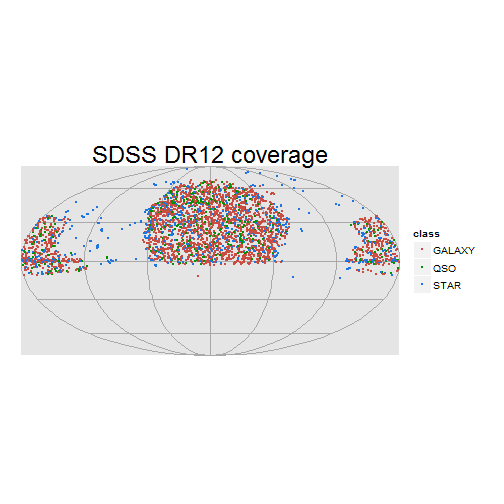# SDSS DR12 - Fetching data using R

Bart Buelens, 26 March 2015.

www.astrostatistics.org

Data Release 12 (DR12) of the Sloan Digital Sky Survey is now available (since early 2015). We obtain some data using SQL queries executed from within R and conduct an initial exploration of the data.

Data are available from the SDSS SkyServer which contains a lot of information about accessing SDSS data. Of particular relevance is a tutorial on how to fetch data using SQL.

## Fetching data using SQL queries

First we load some libraries used in this document.

``````library(RCurl)
library(ggplot2)
``````

We formulate a SQL query, for example the following.

``````mySqlQuery =
"SELECT objID, htmID, z, ra, dec, cx, cy, cz, class, subClass, type, survey, programname
FROM SpecPhoto
WHERE (htmid*37 & 0x000000000000FFFF) < (65)"
``````

This query will select the listed columns from the SpecPhoto view. The WHERE statement makes a random selection of 0.1% of the data. How this works is explained here.

Very useful is the schema browser giving an overview of all tables and view of the data release. For example, details of the SpecPhoto view are shown here.

The SQL query is executed from within R and returns text in csv format, which can be written to disk:

``````mySqlQuery = gsub(pattern="\n",replacement=" ",x=mySqlQuery)
urlBase = "http://skyserver.sdss.org/dr12/en/tools/search/x_sql.aspx"
X = getForm(urlBase, cmd = mySqlQuery, format = "csv")
write(X,file="SDSSsample.csv")
``````

We now have a data file containg the requested SDSS data. The above need only to be conducted once. Thereafter, the data can be loaded from the local hard disk.

## Initial data exploration

The only aim of this short section is to ascertain that the SQL query has produced sensible data that can be used in other analyses.

Start clean and load the data file:

``````rm(list=ls()) # clear everything in the workspace
``````

We now have data frame A containing our data. Let's look at what we have:

``````str(A)
``````
``````'data.frame':   3397 obs. of  13 variables:
\$ objID      : num  1.24e+18 1.24e+18 1.24e+18 1.24e+18 1.24e+18 ...
\$ htmID      : num  1.47e+13 1.63e+13 1.64e+13 1.59e+13 1.65e+13 ...
\$ z          : num  7.72e-02 2.25e-06 7.74e-05 1.11e-01 1.10e-01 ...
\$ ra         : num  209 141 150 171 128 ...
\$ dec        : num  60.8 10 52.5 66.8 28.4 ...
\$ cx         : num  -0.427 -0.768 -0.526 -0.389 -0.545 ...
\$ cy         : num  -0.2379 0.6163 0.3045 0.0635 0.6898 ...
\$ cz         : num  0.873 0.174 0.794 0.919 0.476 ...
\$ class      : Factor w/ 3 levels "GALAXY","QSO",..: 1 3 3 1 1 1 1 1 1 1 ...
\$ subClass   : Factor w/ 93 levels "","A0","A0p",..: 89 59 69 89 1 1 1 1 1 1 ...
\$ type       : int  3 6 6 3 3 3 3 3 3 3 ...
\$ survey     : Factor w/ 4 levels "boss","sdss",..: 2 4 4 2 2 2 2 1 2 2 ...
\$ programname: Factor w/ 38 levels "boss","boss_lofar",..: 7 29 29 7 7 7 7 1 7 7 ...
``````
``````xtabs(~class, A)
``````
``````class
GALAXY    QSO   STAR
2173    454    770
``````
``````xtabs(~survey, A)
``````
``````survey
boss   sdss segue1 segue2
1969   1111    217    100
``````
``````xtabs(~survey+class, A)
``````
``````        class
survey   GALAXY  QSO STAR
boss     1317  364  288
sdss      848   83  180
segue1      3    5  209
segue2      5    2   93
``````

Plot positions on celestial sphere using the Mollweide projection. Colour code objects by their class: galaxy, quasar or star.

``````ggplot() +
geom_point(data=A, aes(x=ra, y=dec, colour=class), size=1) +
scale_colour_hue(l=50) +
coord_map("mollweide",xlim=c(0,360),ylim=c(-90,90)) +
scale_y_continuous(breaks = seq(-90, 90, 30)) +
scale_x_continuous(breaks = seq(0, 360, 60)) +
theme(axis.title = element_blank()) +
theme(axis.ticks = element_blank(), axis.text = element_blank()) +
theme(panel.border = element_blank()) +
theme(panel.grid.major = element_line(colour="darkgrey")) +
ggtitle("SDSS DR12 coverage") +
theme(plot.title = element_text(size = rel(2)))
``````